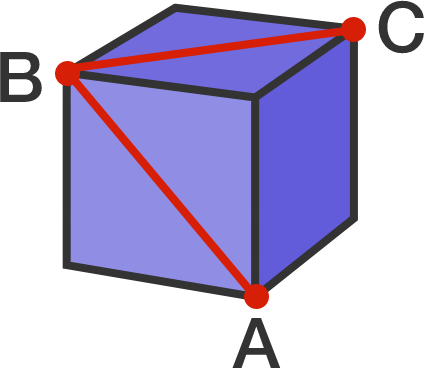# 47 of 100: A Simple Angle

Geometry Level 1A three-dimensional cube is shown above. What is the measure of $\angle ABC$ in degrees?There are a lot of ways to solve this puzzle; look for a fast one, then try to find a second technique!

×